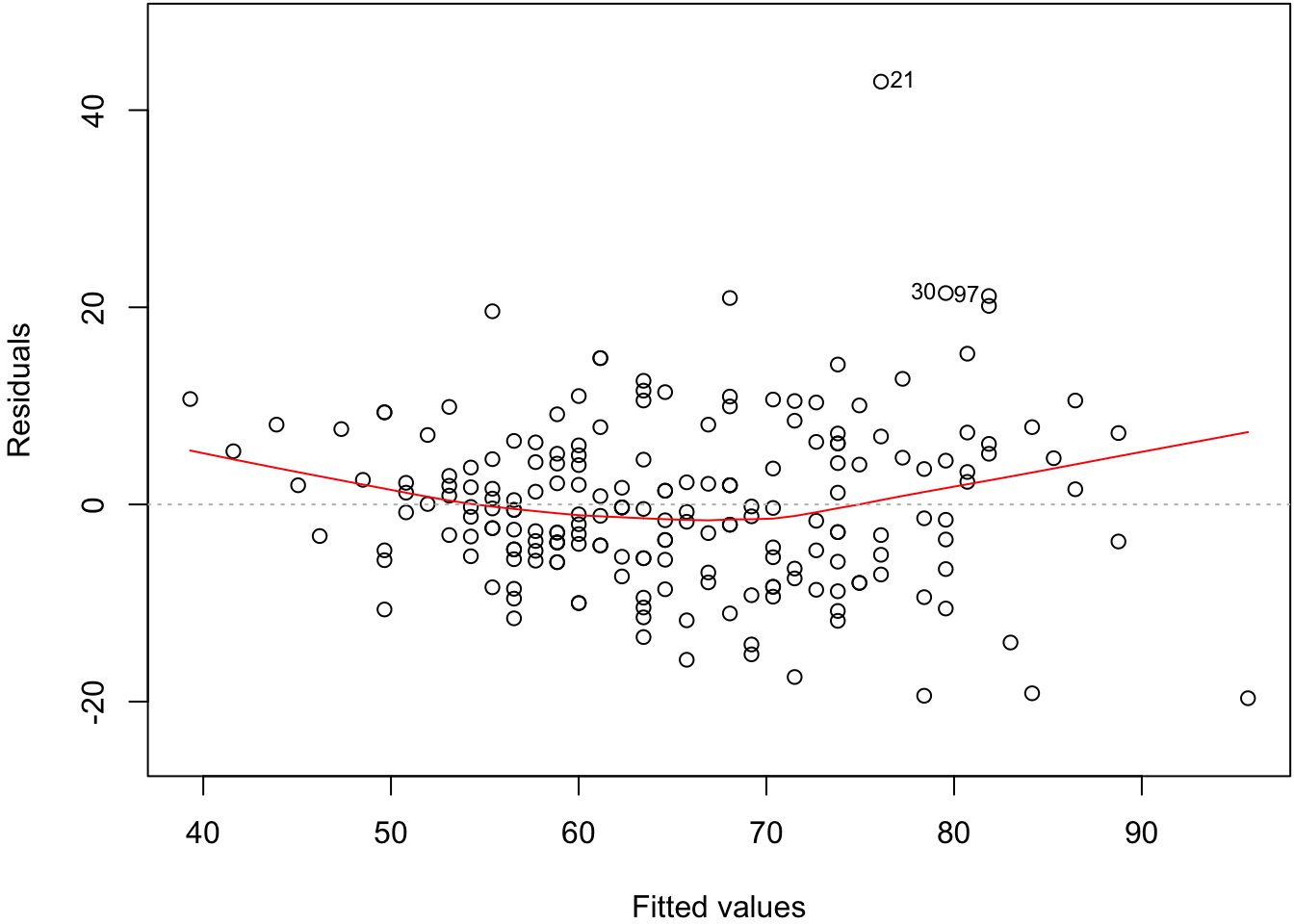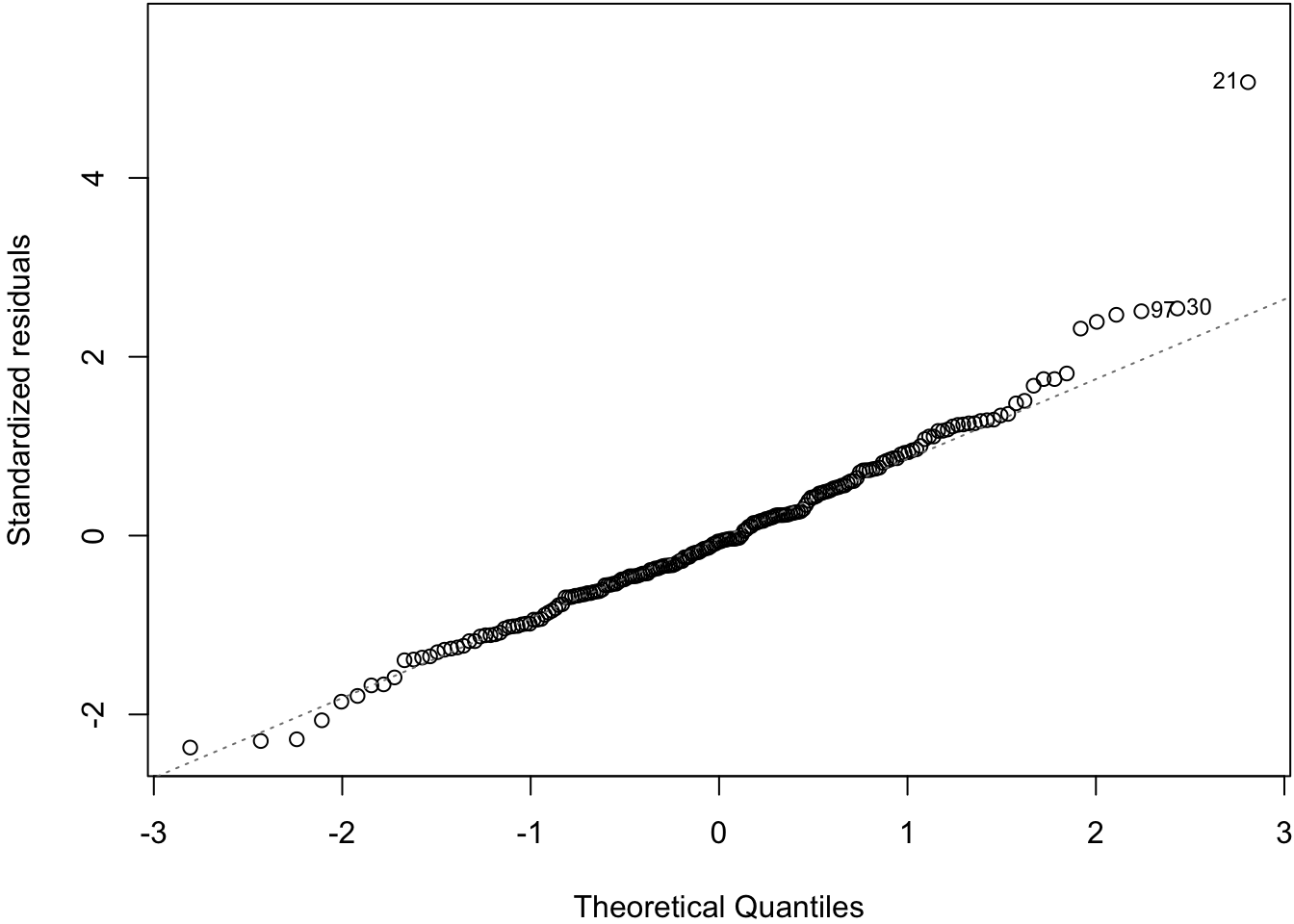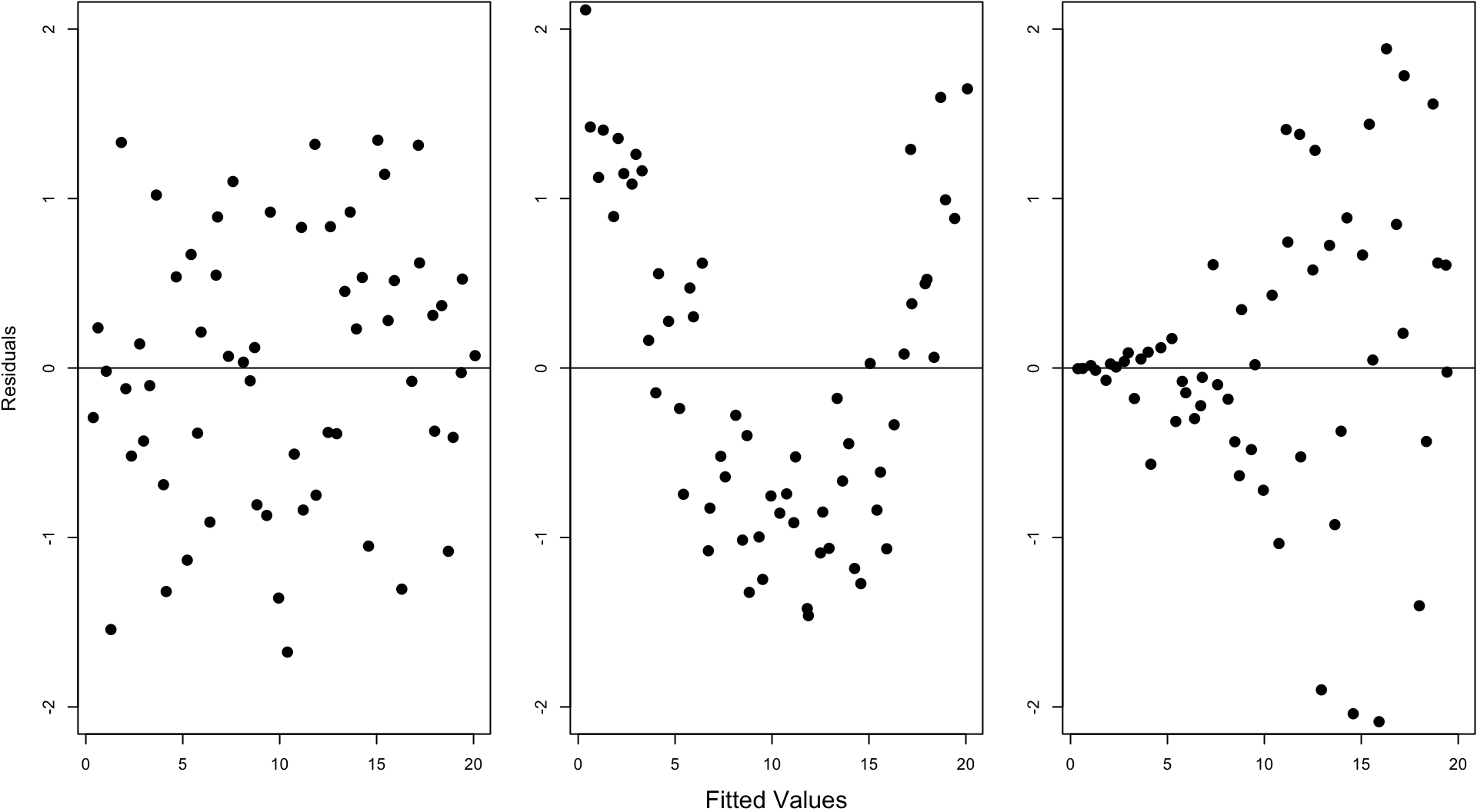# 57lm() Function in R

## 57.1 Calculate the Line in R

The syntax for a model in R is

response variable ~ explanatory variables

where the explanatory variables component can involve several types of terms.

> myfit <- lm(weight ~ height, data=htwt)
> myfit

Call:
lm(formula = weight ~ height, data = htwt)

Coefficients:
(Intercept)       height
-130.91         1.15  

## 57.2 An lm Object is a List

> class(myfit)
 "lm"
> is.list(myfit)
 TRUE
> names(myfit)
 "coefficients"  "residuals"     "effects"       "rank"
 "fitted.values" "assign"        "qr"            "df.residual"
 "xlevels"       "call"          "terms"         "model"        

## 57.3 From the R Help

lm returns an object of class “lm” or for multiple responses of class c(“mlm”, “lm”).

The functions summary and anova are used to obtain and print a summary and analysis of variance table of the results. The generic accessor functions coefficients, effects, fitted.values and residuals extract various useful features of the value returned by lm.

## 57.4 Some of the List Items

These are some useful items to access from the lm object:

• coefficients: a named vector of coefficients
• residuals: the residuals, that is response minus fitted values.
• fitted.values: the fitted mean values.
• df.residual: the residual degrees of freedom.
• call: the matched call.
• model: if requested (the default), the model frame used.

## 57.5summary()

> summary(myfit)

Call:
lm(formula = weight ~ height, data = htwt)

Residuals:
Min      1Q  Median      3Q     Max
-19.658  -5.381  -0.555   4.807  42.894

Coefficients:
Estimate Std. Error t value Pr(>|t|)
(Intercept) -130.91040   11.52792  -11.36   <2e-16 ***
height         1.15009    0.06749   17.04   <2e-16 ***
---
Signif. codes:  0 '***' 0.001 '**' 0.01 '*' 0.05 '.' 0.1 ' ' 1

Residual standard error: 8.505 on 198 degrees of freedom
Multiple R-squared:  0.5946,    Adjusted R-squared:  0.5925
F-statistic: 290.4 on 1 and 198 DF,  p-value: < 2.2e-16

## 57.6summary() List Elements

> mysummary <- summary(myfit)
> names(mysummary)
 "call"          "terms"         "residuals"     "coefficients"
 "aliased"       "sigma"         "df"            "r.squared"
 "adj.r.squared" "fstatistic"    "cov.unscaled" 

## 57.7 Using tidy()

> library(broom)
> tidy(myfit)
# A tibble: 2 x 5
term        estimate std.error statistic  p.value
<chr>          <dbl>     <dbl>     <dbl>    <dbl>
1 (Intercept)  -131.     11.5        -11.4 2.44e-23
2 height          1.15    0.0675      17.0 1.12e-40

## 57.8 Proportion of Variation Explained

The proportion of variance explained by the fitted model is called $$R^2$$ or $$r^2$$. It is calculated by:

$r^2 = \frac{s^2_{\hat{y}}}{s^2_{y}}$

> summary(myfit)$r.squared  0.5945555 > > var(myfit$fitted.values)/var(htwt\$weight)
 0.5945555

## 57.9 Assumptions to Verify

The assumptions on the above linear model are really about the joint distribution of the residuals, which are not directly observed. On data, we try to verify:

1. The fitted values and the residuals show no trends with respect to each other
2. The residuals are distributed approximately Normal$$(0, \sigma^2)$$
3. There are no lurking variables

There are two plots we will use in this course to investigate the first two.

## 57.10 Residual Distribution

> plot(myfit, which=1)## 57.11 Normal Residuals Check

> plot(myfit, which=2)## 57.12 Fitted Values Vs. Obs. Residuals